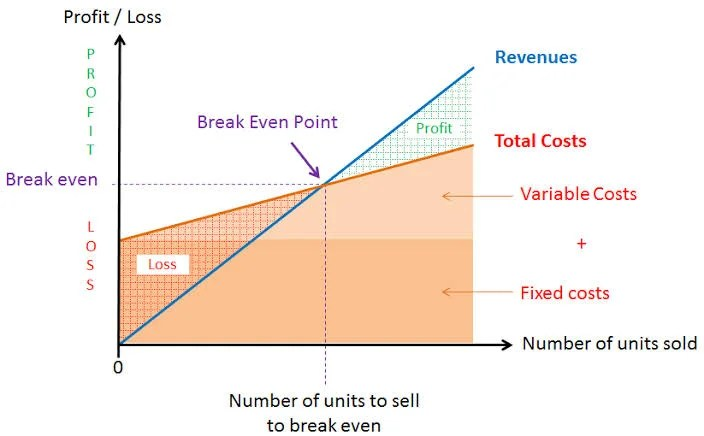# Break Even Point: Meaning, Features and Significance

7th February 2020

Break-even point represents that volume of production where total costs equal to total sales revenue resulting into a no-profit no-loss situation.

In simple words, the break-even point can be defined as a point where total costs (expenses) and total sales (revenue) are equal. Break-even point can be described as a point where there is no net profit or loss. The firm just “breaks even.” Any company which wants to make abnormal profit, desires to have a break-even point. Graphically, it is the point where the total cost and the total revenue curves meet.

Calculation (formula)

Break-even point is the number of units (N) produced which make zero profit.

Revenue – Total costs = 0

Total costs = Variable costs * N + Fixed costs

Revenue = Price per unit * N

Price per unit * N – (Variable costs * N + Fixed costs) = 0

So, break-even point (N) is equal

N = Fixed costs / (Price per unit – Variable costs)The origins of break-even point can be found in the economic concepts of “the point of indifference.” Calculating the break-even point of a company has proved to be a simple but quantitative tool for the managers. The break-even analysis, in its simplest form, facilitates an insight into the fact about revenue from a product or service incorporates the ability to cover the relevant production cost of that particular product or service or not. Moreover, the break-even point is also helpful to managers as the provided info can be used in making important decisions in business, for example preparing competitive bids, setting prices, and applying for loans.

Adding more to the point, break-even analysis is a simple tool defining the lowest quantity of sales which will include both variable and fixed costs. Moreover, such analysis facilitates the managers with a quantity which can be used to evaluate the future demand. If, in case, the break-even point lies above the estimated demand, reflecting a loss on the product, the manager can use this info for taking various decisions. He might choose to discontinue the product, or improve the advertising strategies, or even re-price the product to increase demand.

Another important usage of the break-even point is that it is helpful in recognizing the relevance of fixed and variable cost. The fixed cost is less with a more flexible personnel and equipment thereby resulting in a lower break-even point. The importance of break-even point, therefore, cannot be overstated for a sound business and decision making.

However, the applicability of break-even analysis is affected by numerous assumptions. A violation of these assumptions might result in erroneous conclusions.

### Features of Break Even Point

(i) It helps in the determination of selling price which will give the desired profits.

(ii) It helps in the fixation of sales volume to cover a given return on capital employed.

(iii) It helps in forecasting costs and profit as a result of change in volume.

(iv) It gives suggestions for shift in sales mix.

(v) It helps in making inter-firm comparison of profitability.

(vi) It helps in determination of costs and revenue at various levels of output.

(vii) It is an aid in management decision-making (e.g., make or buy, introducing a product etc.), forecasting, long-term planning and maintaining profitability.

(viii) It reveals business strength and profit earning capacity of a concern without much difficulty and effort.

### Significance of Break Even Point

(i) All costs can be separated into fixed and variable components

(ii) Fixed costs will remain constant at all volumes of output

(iii) Variable costs will fluctuate in direct proportion to volume of output

(iv) Selling price will remain constant

(v) Product-mix will remain unchanged

(vi) The number of units of sales will coincide with the units produced so that there is no opening or closing stock

(vii) Productivity per worker will remain unchanged

(viii) There will be no change in the general price level

### Limitations of Break-Even Analysis

1. Break-even analysis is based on the assumption that all costs and expenses can be clearly separated into fixed and variable components. In practice, however, it may not be possible to achieve a clear-cut division of costs into fixed and variable types.
2. It assumes that fixed costs remain constant at all levels of activity. It should be noted that fixed costs tend to vary beyond a certain level of activity.
3. It assumes that variable costs vary proportionately with the volume of output. In practice, they move, no doubt, in sympathy with volume of output, but not necessarily in direct proportions..
4. The assumption that selling price remains unchanged gives a straight revenue line which may not be true. Selling price of a product depends upon certain factors like market demand and supply, competition etc., so it, too, hardly remains constant.
5. The assumption that only one product is produced or that product mix will remain unchanged is difficult to find in practice.
6. Apportionment of fixed cost over a variety of products poses a problem.
7. It assumes that the business conditions may not change which is not true.
8. It assumes that production and sales quantities are equal and there will be no change in opening and closing stock of finished product, these do not hold good in practice.
9. The break-even analysis does not take into consideration the amount of capital employed in the business. In fact, capital employed is an important determinant of the profitability of a concern.